# RS Aggarwal Solutions for Class 7 Maths Exercise 17C Chapter 17 Constructions

RS Aggarwal Solutions for Class 7 Maths Exercise 17C Chapter 17 Constructions in simple PDF are available here. This exercise is of completely objective type questions. It includes all topics present in the RS Aggarwal Solutions for Class 7 Maths Chapter 17 Constructions. After solving these objective type questions, students can absorb more knowledge from the chapter and consequently, score high-grades in Maths.

## Download the PDF of RS Aggarwal Solutions For Class 7 Maths Chapter 17 Constructions – Exercise 17C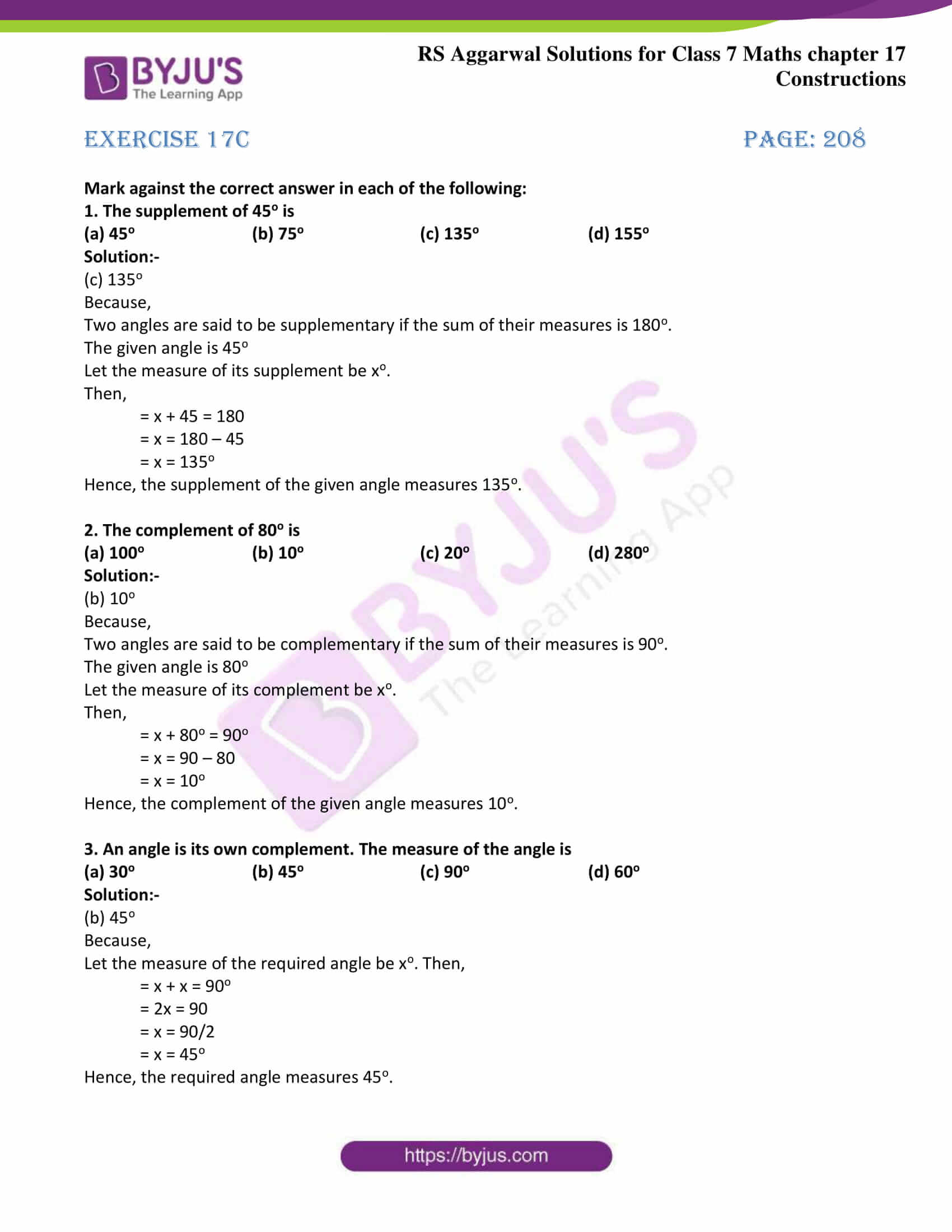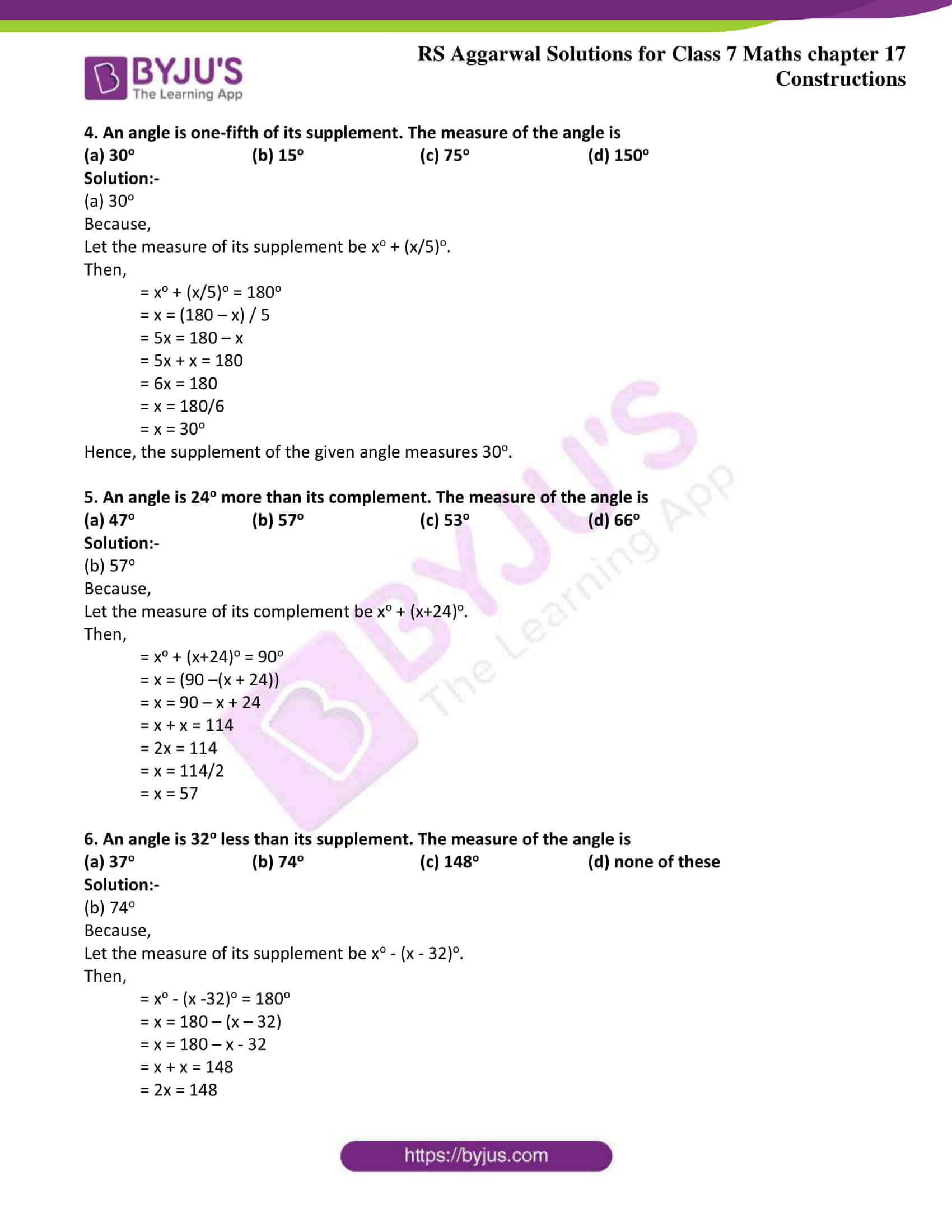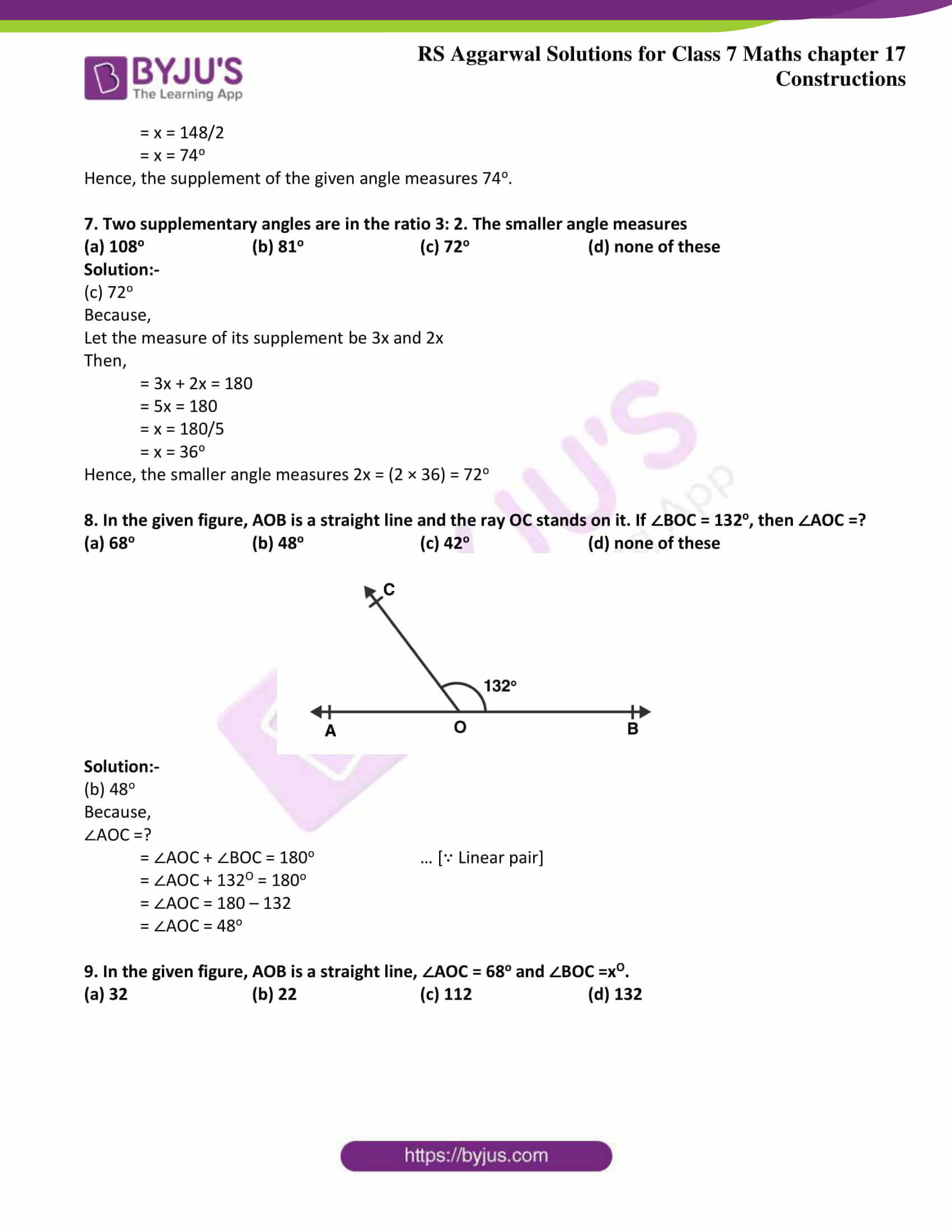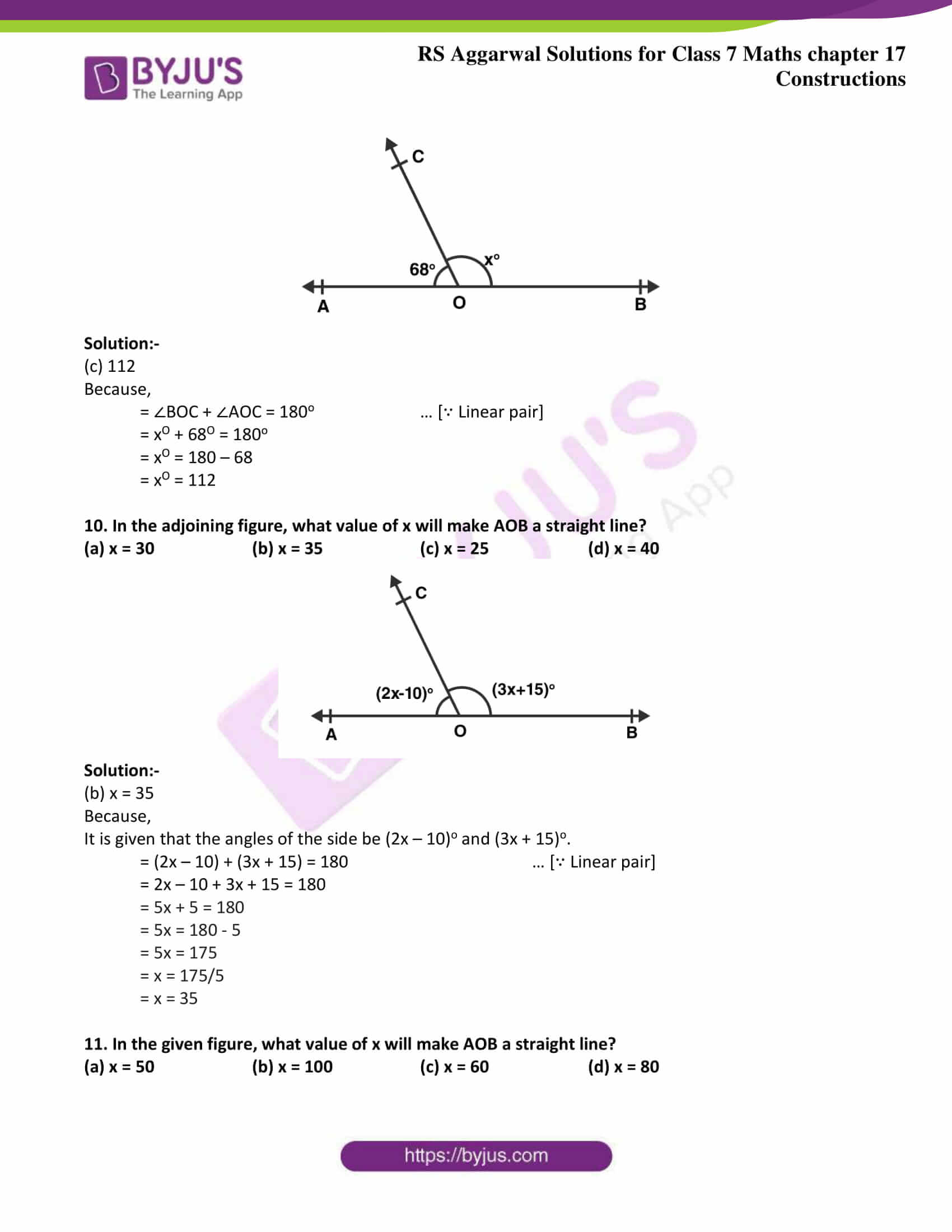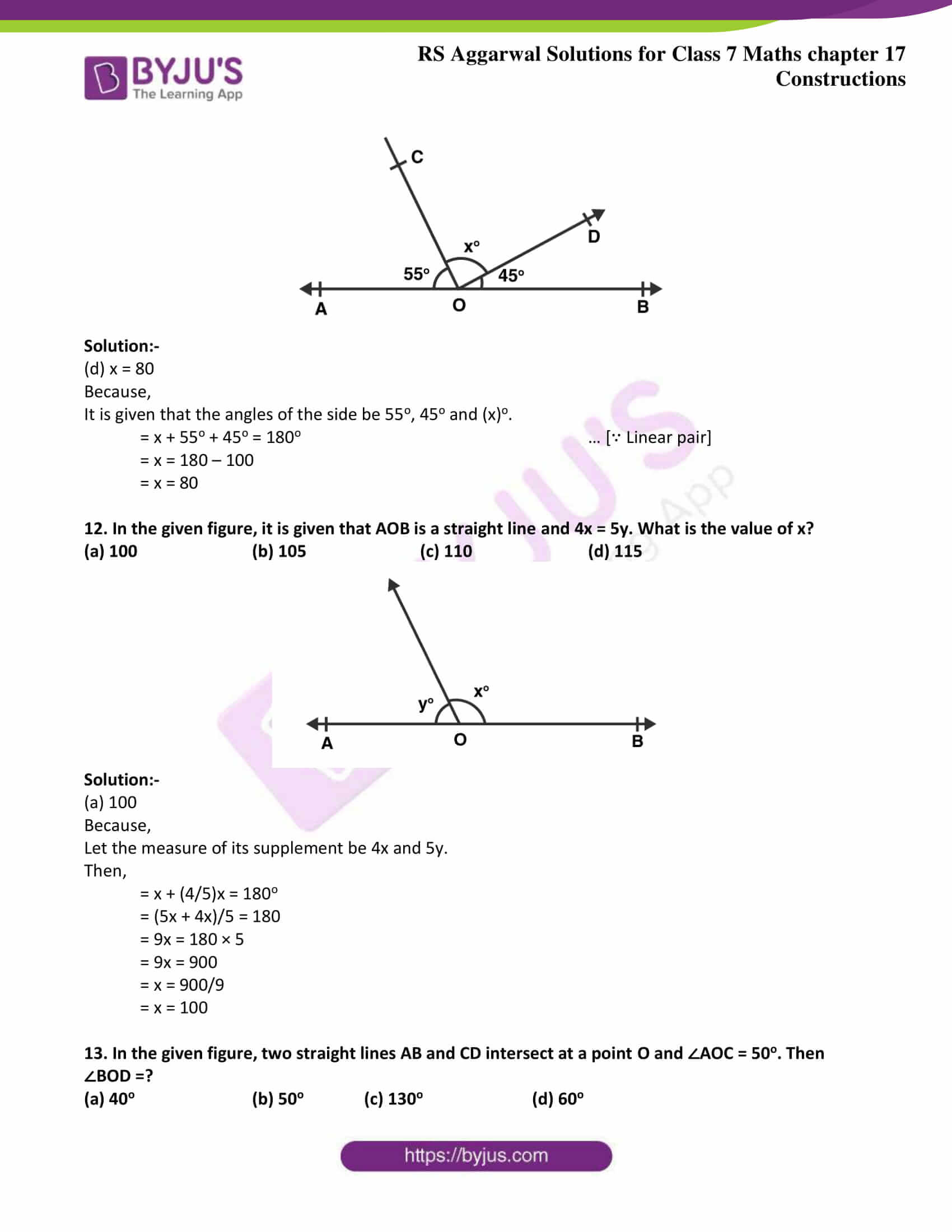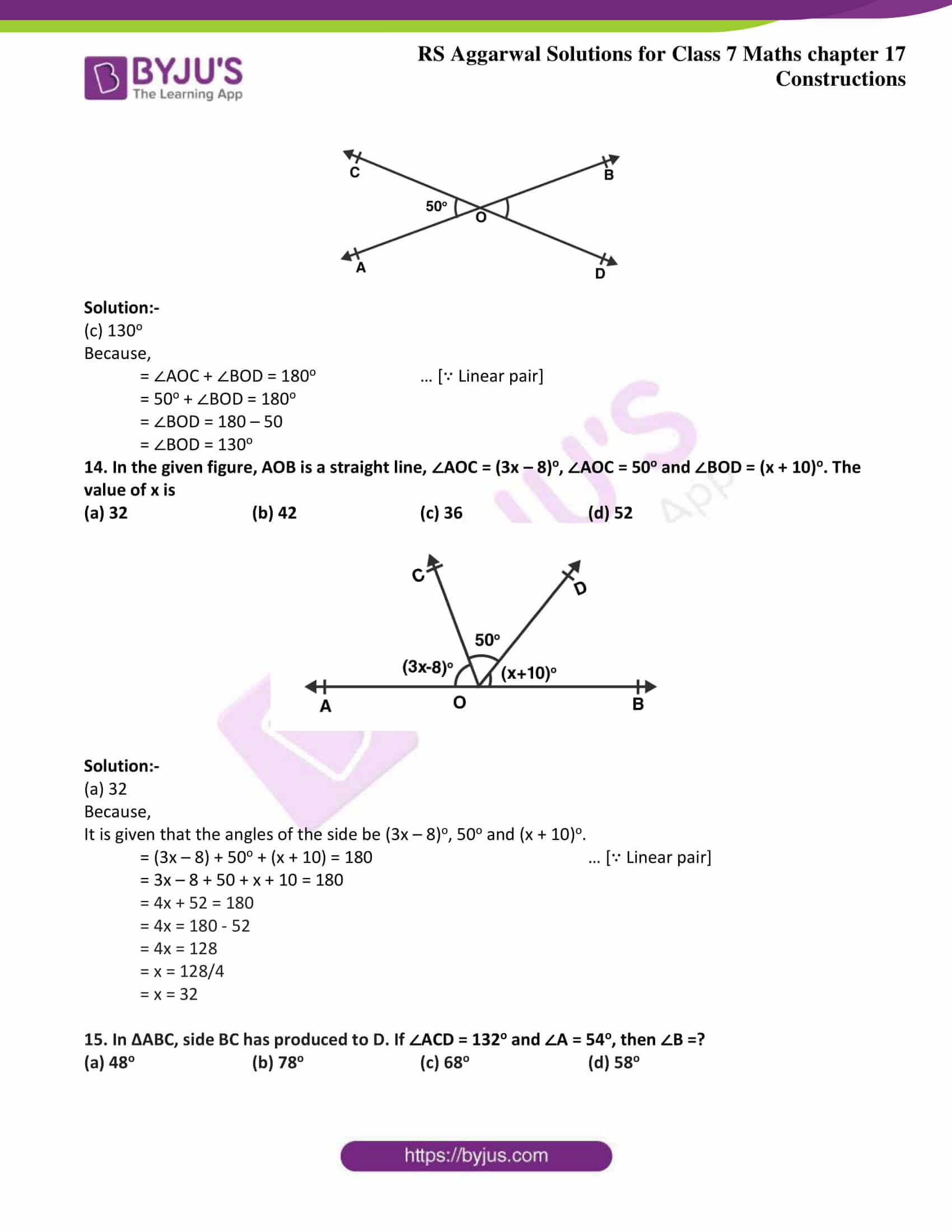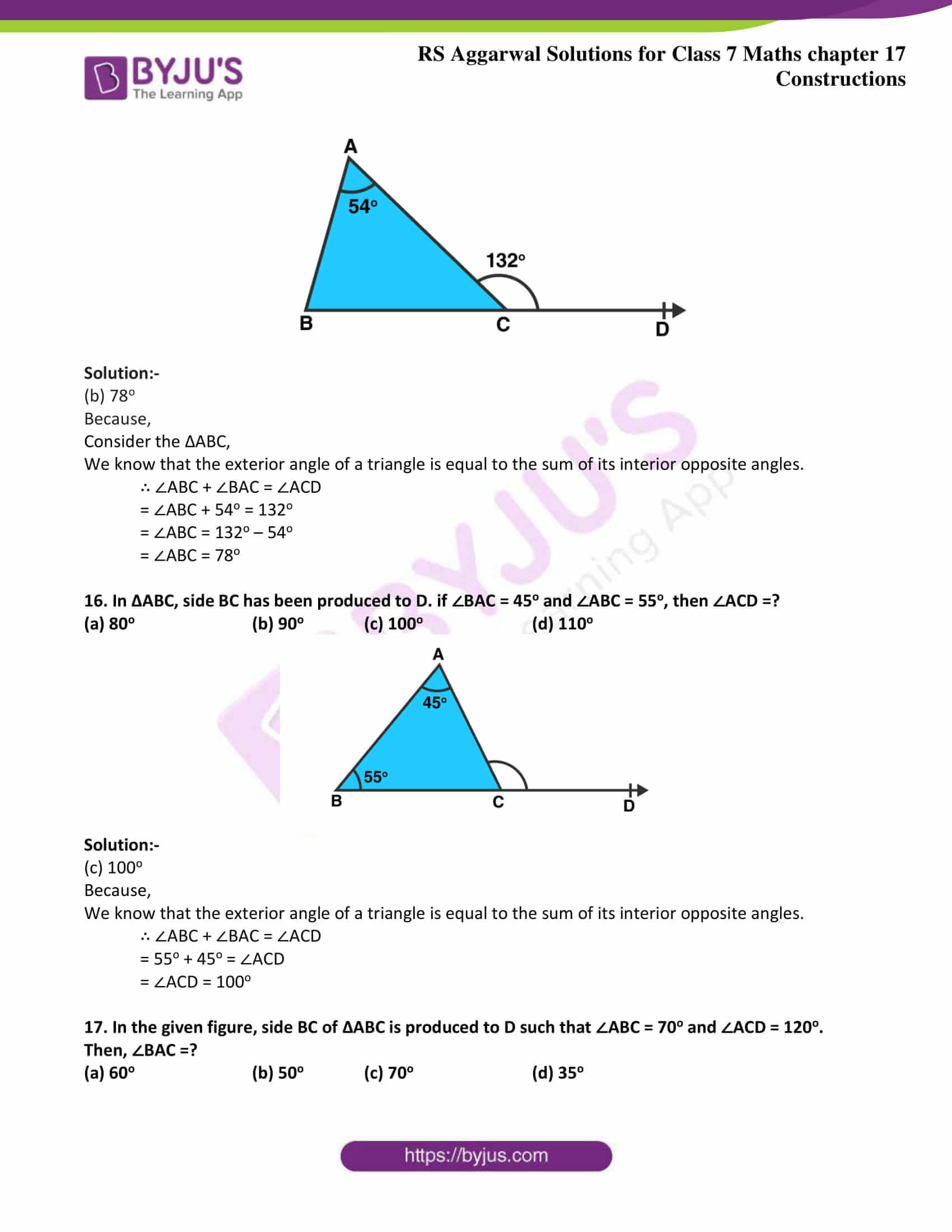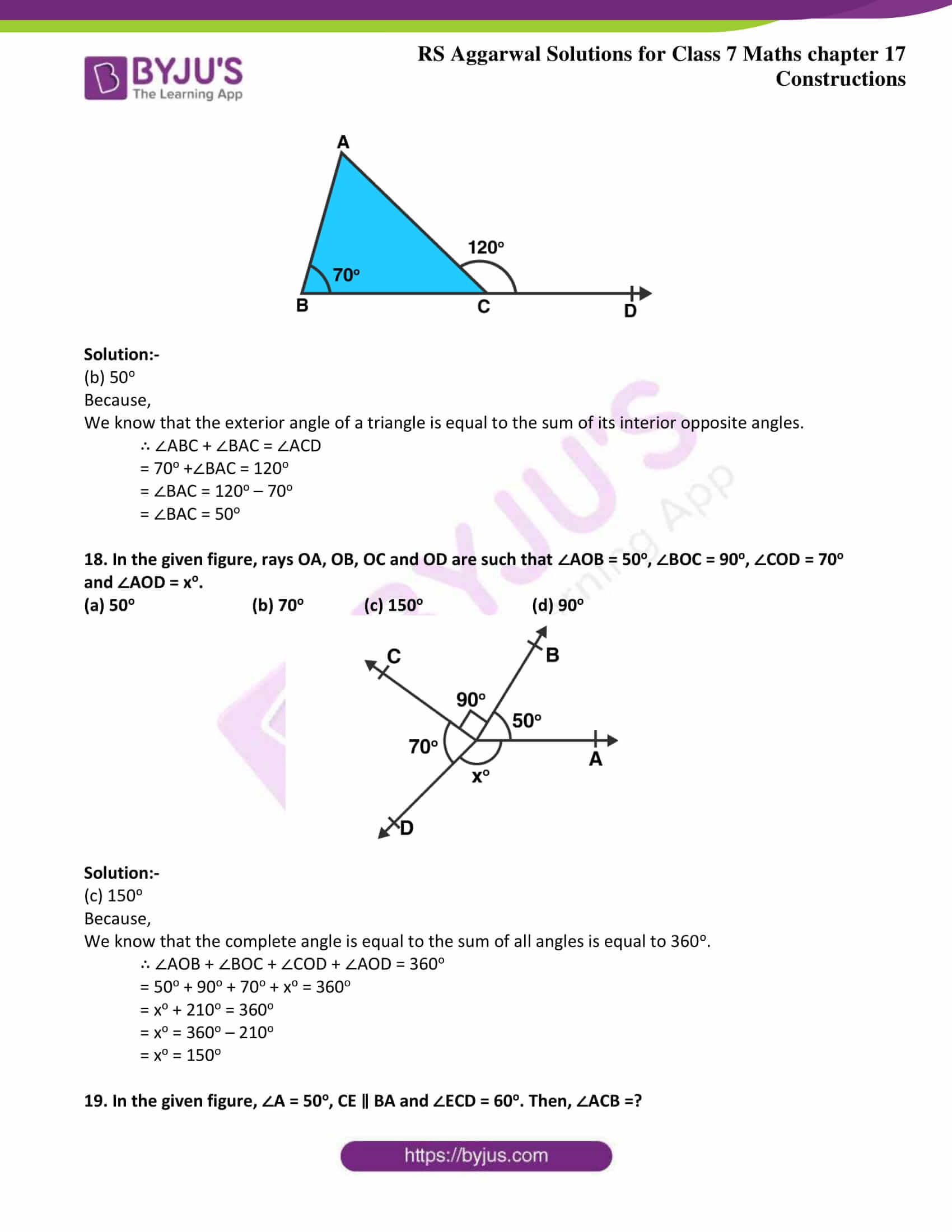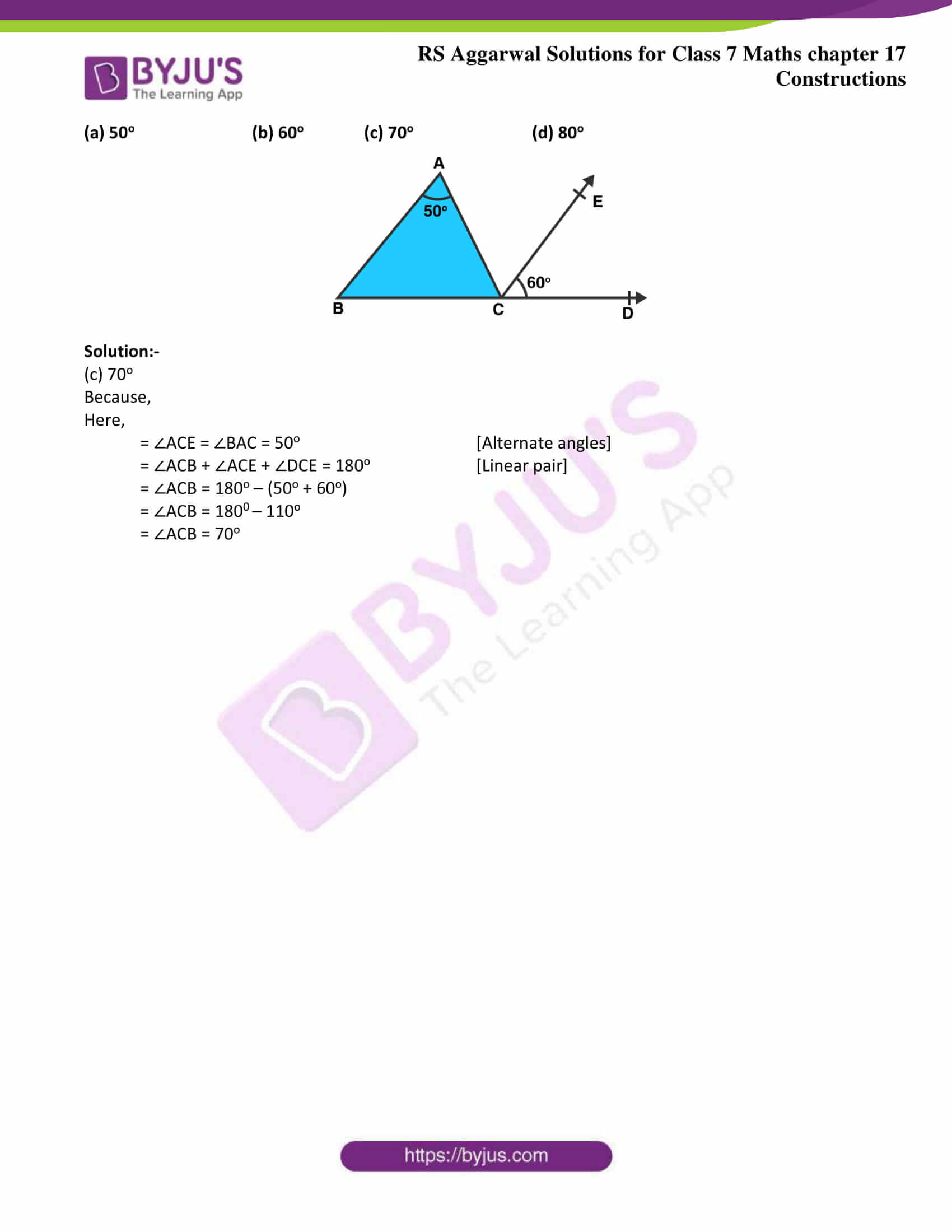### Access answers to Maths RS Aggarwal Solutions for Class 7 Chapter 17 – Constructions Exercise 17C

Mark against the correct answer in each of the following:

1. The supplement of 45o is

(a) 45o (b) 75o (c) 135o (d) 155o

Solution:-

(c) 135o

Because,

Two angles are said to be supplementary if the sum of their measures is 180o.

The given angle is 45o

Let the measure of its supplement be xo.

Then,

= x + 45 = 180

= x = 180 – 45

= x = 135o

Hence, the supplement of the given angle measures 135o.

2. The complement of 80o is

(a) 100o (b) 10o (c) 20o (d) 280o

Solution:-

(b) 10o

Because,

Two angles are said to be complementary if the sum of their measures is 90o.

The given angle is 80o

Let the measure of its complement be xo.

Then,

= x + 80o = 90o

= x = 90 – 80

= x = 10o

Hence, the complement of the given angle measures 10o.

3. An angle is its own complement. The measure of the angle is

(a) 30o (b) 45o (c) 90o (d) 60o

Solution:-

(b) 45o

Because,

Let the measure of the required angle be xo. Then,

= x + x = 90o

= 2x = 90

= x = 90/2

= x = 45o

Hence, the required angle measures 45o.

4. An angle is one-fifth of its supplement. The measure of the angle is

(a) 30o (b) 15o (c) 75o (d) 150o

Solution:-

(a) 30o

Because,

Let the measure of its supplement be xo + (x/5)o.

Then,

= xo + (x/5)o = 180o

= x = (180 – x) / 5

= 5x = 180 – x

= 5x + x = 180

= 6x = 180

= x = 180/6

= x = 30o

Hence, the supplement of the given angle measures 30o.

5. An angle is 24o more than its complement. The measure of the angle is

(a) 47o (b) 57o (c) 53o (d) 66o

Solution:-

(b) 57o

Because,

Let the measure of its complement be xo + (x+24)o.

Then,

= xo + (x+24)o = 90o

= x = (90 –(x + 24))

= x = 90 – x + 24

= x + x = 114

= 2x = 114

= x = 114/2

= x = 57

6. An angle is 32o less than its supplement. The measure of the angle is

(a) 37o (b) 74o (c) 148o (d) none of these

Solution:-

(b) 74o

Because,

Let the measure of its supplement be xo – (x – 32)o.

Then,

= xo – (x -32)o = 180o

= x = 180 – (x – 32)

= x = 180 – x – 32

= x + x = 148

= 2x = 148

= x = 148/2

= x = 74o

Hence, the supplement of the given angle measures 74o.

7. Two supplementary angles are in the ratio 3: 2. The smaller angle measures

(a) 108o (b) 81o (c) 72o (d) none of these

Solution:-

(c) 72o

Because,

Let the measure of its supplement be 3x and 2x

Then,

= 3x + 2x = 180

= 5x = 180

= x = 180/5

= x = 36o

Hence, the smaller angle measures 2x = (2 × 36) = 72o

8. In the given figure, AOB is a straight line and the ray OC stands on it. If ∠BOC = 132o, then ∠AOC =?

(a) 68o (b) 48o (c) 42o (d) none of these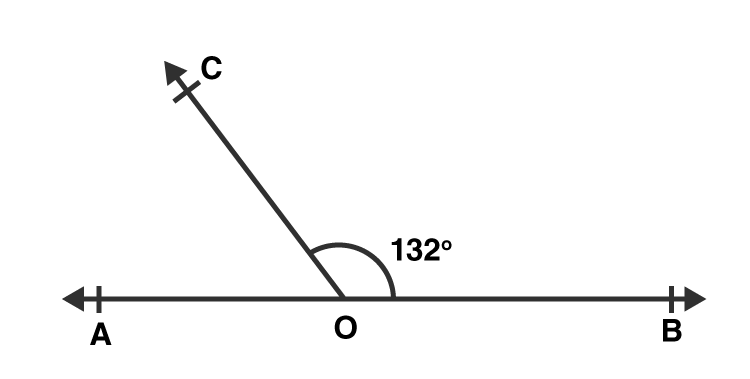Solution:-

(b) 48o

Because,

∠AOC =?

= ∠AOC + ∠BOC = 180o … [∵ Linear pair]

= ∠AOC + 132O = 180o

= ∠AOC = 180 – 132

= ∠AOC = 48o

9. In the given figure, AOB is a straight line, ∠AOC = 68o and ∠BOC =xO.

(a) 32 (b) 22 (c) 112 (d) 132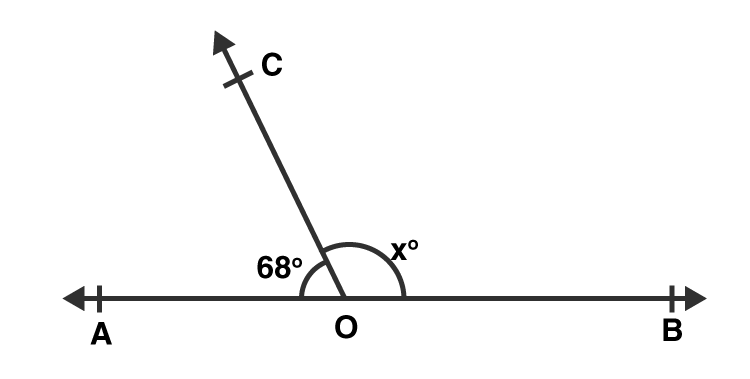Solution:-

(c) 112

Because,

= ∠BOC + ∠AOC = 180o … [∵ Linear pair]

= xO + 68O = 180o

= xO = 180 – 68

= xO = 112

10. In the adjoining figure, what value of x will make AOB a straight line?

(a) x = 30 (b) x = 35 (c) x = 25 (d) x = 40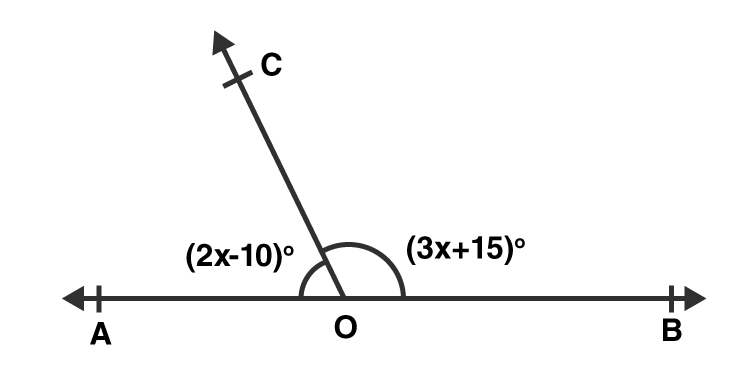Solution:-

(b) x = 35

Because,

It is given that the angles of the side be (2x – 10)o and (3x + 15)o.

= (2x – 10) + (3x + 15) = 180 … [∵ Linear pair]

= 2x – 10 + 3x + 15 = 180

= 5x + 5 = 180

= 5x = 180 – 5

= 5x = 175

= x = 175/5

= x = 35

11. In the given figure, what value of x will make AOB a straight line?

(a) x = 50 (b) x = 100 (c) x = 60 (d) x = 80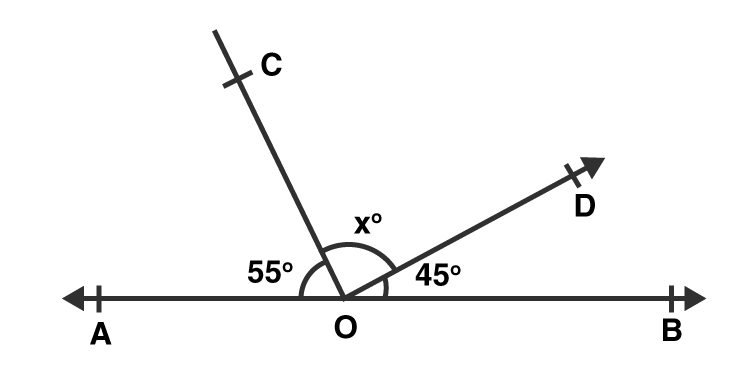Solution:-

(d) x = 80

Because,

It is given that the angles of the side be 55o, 45o and (x)o.

= x + 55o + 45o = 180o … [∵ Linear pair]

= x = 180 – 100

= x = 80

12. In the given figure, it is given that AOB is a straight line and 4x = 5y. What is the value of x?

(a) 100 (b) 105 (c) 110 (d) 115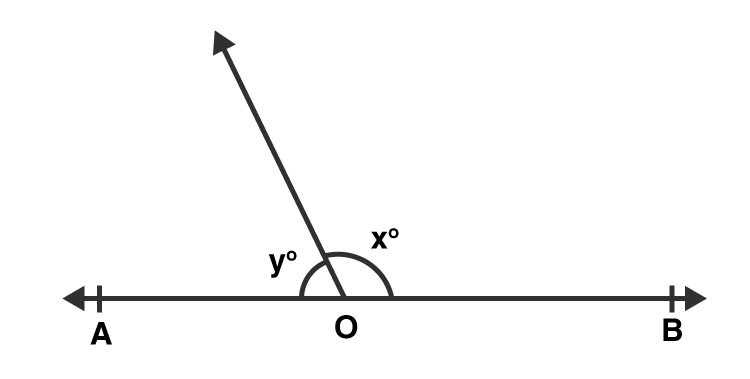Solution:-

(a) 100

Because,

Let the measure of its supplement be 4x and 5y.

Then,

= x + (4/5)x = 180o

= (5x + 4x)/5 = 180

= 9x = 180 × 5

= 9x = 900

= x = 900/9

= x = 100

13. In the given figure, two straight lines AB and CD intersect at a point O and ∠AOC = 50o. Then ∠BOD =?

(a) 40o (b) 50o (c) 130o (d) 60o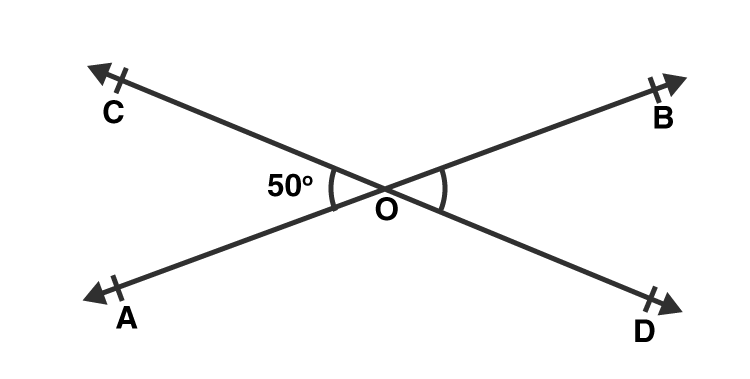Solution:-

(c) 130o

Because,

= ∠AOC + ∠BOD = 180o … [∵ Linear pair]

= 50o + ∠BOD = 180o

= ∠BOD = 180 – 50

= ∠BOD = 130o

14. In the given figure, AOB is a straight line, ∠AOC = (3x – 8)o, ∠AOC = 50o and ∠BOD = (x + 10)o. The value of x is

(a) 32 (b) 42 (c) 36 (d) 52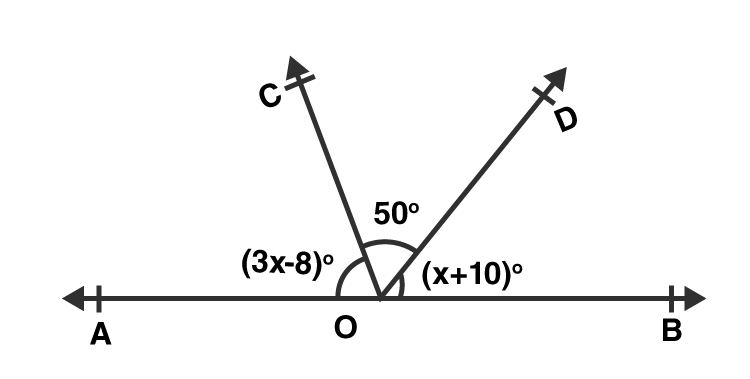Solution:-

(a) 32

Because,

It is given that the angles of the side be (3x – 8)o, 50o and (x + 10)o.

= (3x – 8) + 50o + (x + 10) = 180 … [∵ Linear pair]

= 3x – 8 + 50 + x + 10 = 180

= 4x + 52 = 180

= 4x = 180 – 52

= 4x = 128

= x = 128/4

= x = 32

15. In ΔABC, side BC has produced to D. If ∠ACD = 132o and ∠A = 54o, then ∠B =?

(a) 48o (b) 78o (c) 68o (d) 58o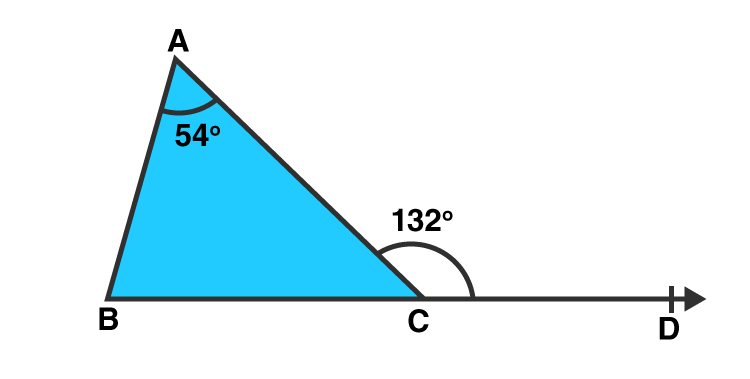Solution:-

(b) 78o

Because,

Consider the ΔABC,

We know that the exterior angle of a triangle is equal to the sum of its interior opposite angles.

∴ ∠ABC + ∠BAC = ∠ACD

= ∠ABC + 54o = 132o

= ∠ABC = 132o – 54o

= ∠ABC = 78o

16. In ΔABC, side BC has been produced to D. if ∠BAC = 45o and ∠ABC = 55o, then ∠ACD =?

(a) 80o (b) 90o (c) 100o (d) 110o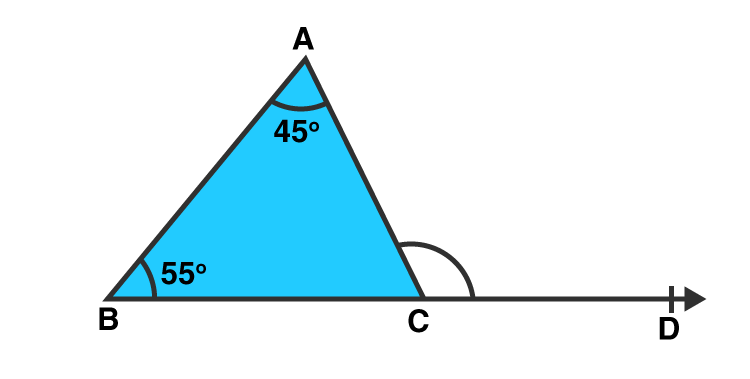Solution:-

(c) 100o

Because,

We know that the exterior angle of a triangle is equal to the sum of its interior opposite angles.

∴ ∠ABC + ∠BAC = ∠ACD

= 55o + 45o = ∠ACD

= ∠ACD = 100o

17. In the given figure, side BC of ΔABC is produced to D such that ∠ABC = 70o and ∠ACD = 120o. Then, ∠BAC =?

(a) 60o (b) 50o (c) 70o (d) 35o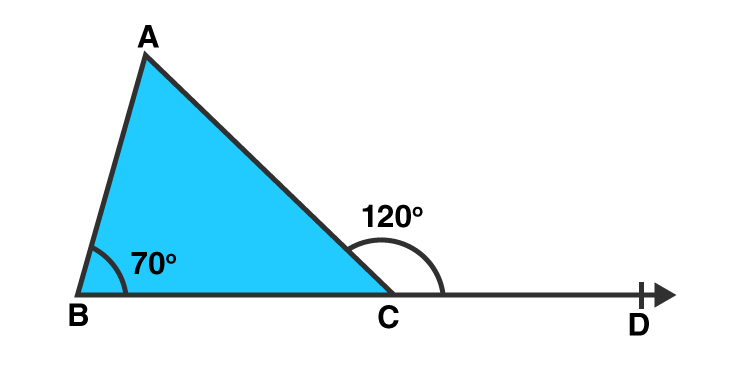Solution:-

(b) 50o

Because,

We know that the exterior angle of a triangle is equal to the sum of its interior opposite angles.

∴ ∠ABC + ∠BAC = ∠ACD

= 70o +∠BAC = 120o

= ∠BAC = 120o – 70o

= ∠BAC = 50o

18. In the given figure, rays OA, OB, OC and OD are such that ∠AOB = 50o, ∠BOC = 90o, ∠COD = 70o and ∠AOD = xo.

(a) 50o (b) 70o (c) 150o (d) 90o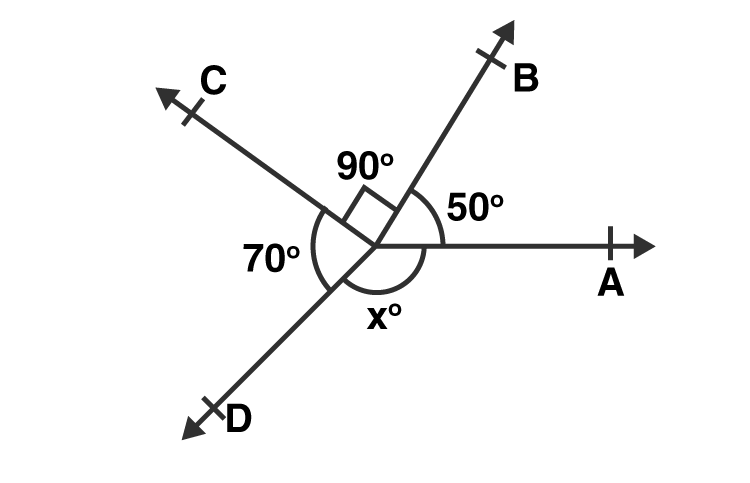Solution:-

(c) 150o

Because,

We know that the complete angle is equal to the sum of all angles is equal to 360o.

∴ ∠AOB + ∠BOC + ∠COD + ∠AOD = 360o

= 50o + 90o + 70o + xo = 360o

= xo + 210o = 360o

= xo = 360o – 210o

= xo = 150o

19. In the given figure, ∠A = 50o, CE ∥ BA and ∠ECD = 60o. Then, ∠ACB =?

(a) 50o (b) 60o (c) 70o (d) 80o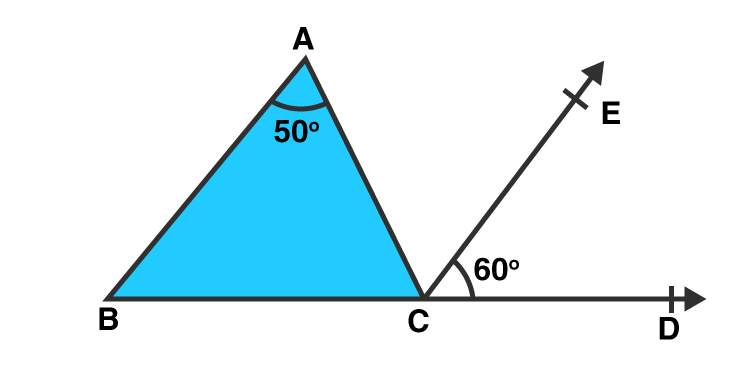Solution:-

(c) 70o

Because,

Here,

= ∠ACE = ∠BAC = 50o [Alternate angles]

= ∠ACB + ∠ACE + ∠DCE = 180o [Linear pair]

= ∠ACB = 180o – (50o + 60o)

= ∠ACB = 1800 – 110o

= ∠ACB = 70o

### Access other exercises of RS Aggarwal Solutions For Class 7 Chapter 17 – Constructions

Exercise 17A Solutions

Exercise 17B Solutions